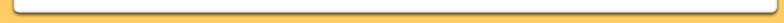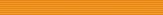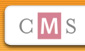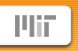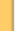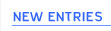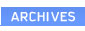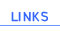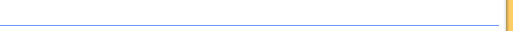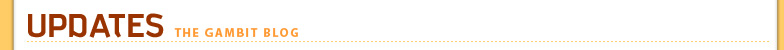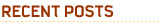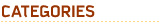Cat Movement (Scaring Cats)

We had several problems arise while working on our Scaring Cats idea. We tried to begin simply by looking at the movement of the cat through an obstacle course. By then, we had already generated a number of objects that the cat could interact with (a bag would attract the cat and cause the cat to slide across the floor, a hairdryer would scare the cat away, etc.) We wanted to be able to model the cat's interaction with both the objects and the player (a dog who could scare the cat in certain directions) in real time. We attempted to do so by using a grid-based game space and allowing the players to alternate movements (as if in a turn-based game). We were forced to decide how fast the cat and dog could move. The cat, we decided, could move at one of three speeds (1 square/ turn, 2 squares, 3 squares) and the dog could only move at the cat's medium speed at the fastest (2 squares). We set up several play-throughs to test this motion, wherein one team member set up an obstacle course (with the goal that the cat would move from point A to B simply by influence from the objects attractiveness/ repellent power) and the others moved the cat and dog. We quickly discovered that we had not defined the cat's interaction with the objects closely enough. Here's an overview of what we found:

The problem with our current play-testing is that there are too many variables that determine whether the cat interacts with the object:

1. Each object has a different radius of interaction AND
2. The nature of this radius depends on the direction from which the cat approaches the object AND
3. The nature of this radius depends on the speed that the cat approaches at

If the interaction occurs at all (unclear) it is then difficult to determine HOW the cat interacts with the object:

1. Does the cat interact at all?
2. Which is the resultant cat vector direction (can depend on speed, entrance angle)?
3. What is the resultant cat magnitude?

Example:

So this means that our bag (which is only accessible from the 'front' and has a radius of the spaces located at (-1,1) (0,1) (1,1) ) has 6 directions from which it can be uniquely approached . [E.g. if that cat approaches from <0 1> it will always enter the bag, but if it comes from <0 -1> it will never enter the bag]. Notice how difficult already it is to describe interactions when the cat is not approaching from one of the 8 primary directions. For instance if the cat is traveling from (1,2) to (1,1) to (1,0), this is completely unique from a diagonal approach and yet had not been included in our original analysis.

The cat can also be traveling at one of three speeds, each of which may have a unique effect on the interaction. E.g. If the cat is to the North East of the box and traveling Westward (different spatial thinking then we were using before, but necessary to describe the movement) then it will enter at (0,1) if traveling at speed 1, (-1,1) if traveling at speed 2, and not at all if traveling speed three. So three speeds, six directions... that means there are 18 unique interactions that must be defined for each object. That's too many for a naive user to be able to just 'pick up on' while trying out the game.
If this seems confusing, that's because it is.

Solutions:

- Should we simplify objects down to basic properties? Similar to the excel sheet we were working on--need simpler objects with less unique properties.
- Should we scrap this idea and move to sinks and sources? May be even harder to model.
- Should we eliminate the ability to move diagonally?
- Should we reduce the number of speeds?
- Should we alter our thinking here--should the cat interact with the SPACES around an object the same way every time (regardless of the direction it entered the space) and maybe only vary based on speed.
o There are a lot of problems with thinking in 'vectors'

 « Zombie Prototype v2 (Weise Zombie Project) Main (Everything I Do) I Do it for You »